﻿ Calculation method > Deygout

# DEYGOUT

Navegación:  Calculation method > Deygout

# DEYGOUT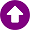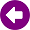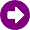DESCRIPTION This model is based on paper by Deygout  and it has been improved by Xirio technical development group with the aim of taking into account several aspects not considered in the first version of the model. The model assumes as a main condition that all obstacles along the path are “knife-edge obstacles”. The calculation of diffraction losses caused by any obstacles number is carried out through a generalized recursive algorithm. As an additional resource, the help file related to is useful, because there are some general concepts about diffraction that can be utilised for a better understanding of Deygout’s model.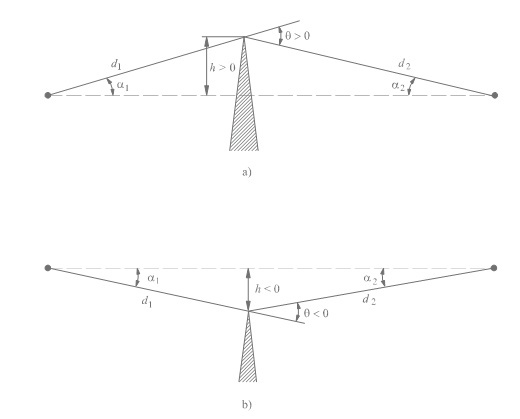Ilustration of an idealized knife-edge obstacle. DEVELOPMENT In the first step of the model, the main obstacle along the path is identified, which is characterized by having the highest value of the diffraction variable Vp. Then, the diffraction losses J (Vp) are calculated. If the direct ray is blocked by the obstacle (Vp > 0), the propagation path is divided into two sub-paths with the two link ends and the obstacle as reference points. For each one of them, the main obstacle is identified, in addition to their respective diffraction variables Vt and Vr; and then the diffraction losses in the sub-path caused by each one of the obstacles, J (Vp) and J (Vr), are calculated. If one of these secondary obstacles blocks the ray (Vt,r > 0) along the sub-path, it is used as a reference point to define two new sub-paths, in a similar way as it was detailed in the previous paragraph. The procedure continues recursively until no obstacle exists blocking the ray in any of the sub-paths. Given a scenario where some obstacles penetrate into the first Fresnel zone, without causing a total blockage situation, the losses of these sub-paths are added to each other (EMP method).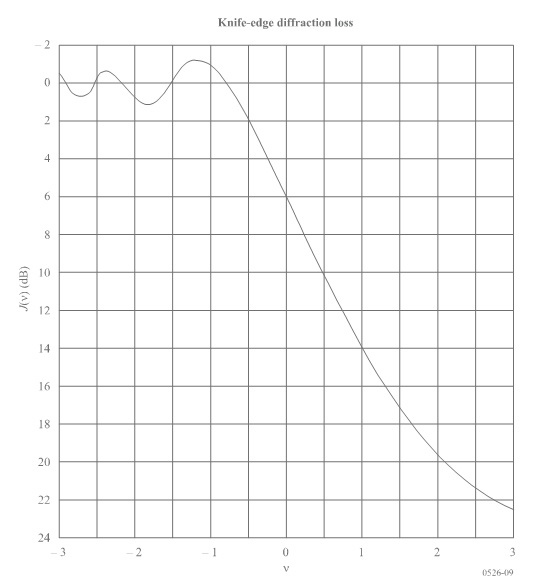Graphical representation of knife-edge diffraction loss. REFERENCES  J. Deygout, "Multiple knife-edge diffraction of microwaves," in IEEE Transactions on Antennas and Propagation, vol. 14, no. 4, pp. 480-489, Jul 1966. doi: 10.1109/TAP.1966.1138719